ZeitschriftendatenFormat
Zeitschrift
eISSN
2300-0929
Erstveröffentlichung
19 Oct 2012
Erscheinungsweise
4 Hefte pro Jahr
Sprachen
EnglischUneingeschränkter Zugang

# Estimation of Seams in Paraglider Wing

###### Seitenbereich: -
ZeitschriftendatenFormat
Zeitschrift
eISSN
2300-0929
Erstveröffentlichung
19 Oct 2012
Erscheinungsweise
4 Hefte pro Jahr
Sprachen
Englisch

The aim of the article is to analyze the seams connecting the covering textiles in paraglider wing and estimate their physical strength. The most extreme flight parameters (the angle of attack and velocity) in the numerical model of the wing allow the determination of the mean pressure inside the contour and the maximal difference between the pressures on the inner and the outer material surfaces. The preprocessing stage and numerical calculations in Ansys environment consider three different covering materials. The tool Porous Media is used to create the objects of a given value of air permeability. The estimated parameter of seam is the maximal pressure difference between both sides of the covering material, which that interacts with the test results. The quality index of the seam is defined by the maximal force to seam rupture and relative elongation during rupture. The obtained results indicate that the seams are resistant to the pressure difference caused by the most extreme flight parameters.

Introduction

The paraglider wing is a flexible structure without stiffening components of the airfoil shape during the flight . The entering vents on the leading edge secure the aerodynamic shape by the suitable air pressure inside the contour. The covering is made of impregnated synthetic textiles; the elements of prescribed properties and dimensions are connected by seams. The seam strength secures the airfoil shape and the air permeability of covering material.

The seams are subjected to various phenomena during the flight, for instance, heat transfer. The physical model determines the textile composite, composed of fabric and seam structure. The mathematical model includes the heat transfer equation and a set of conditions . The impact of seams on heat insulation and moisture resistance is analyzed in a previous paper  for different types of sealed seams, typically used to connect the parts of clothing. The coupled transport is defined by the heat and mass transport equations and a set of conditions [4, 5]. The heat is transported with mass, and the mass transport with heat is negligible. The mass sorption in fibers is simulated as a two-stage phenomenon. The shape of textile structures subjected to diffusion can be optimized using the homogenization and the sensitivity expression . The thermoplastic polymer creates additional protection, improves the aesthetic qualities, and retains the stiffness of laminates against creasing .

The problem can be solved on the microscale using the SolidWorks software in a 3D environment . The method for measuring the surface geometry by laser techniques is applied to define the specific structure and properties, as well as the surface geometry of fabrics [9, 10].

The pressure inside the structure is determined using the numerical model of the wing and the flight parameters, namely, the angle of attack and velocity. The state variable in paraglider flow problems is the pressure distribution inside the contour. The ANSYS environment allows the creation of a finite volume mesh and numerical calculations related to three different covering materials. The ANSYS Fluent is divided into three stages: (i) the preprocessing stage prepares the geometry and numerical mesh;, (ii) the solving simulates the flow of paraglider wing made of permeable materials;, and (iii) the postprocessing stage visualizes the distribution of the state variable.

The main goals of the article are as follows: (i) determining the optimal angle of attack and the velocity of the wing during the flight, as well as the most extreme conditions in respect of the biggest inner forces inside the structure; (ii) specifying the mean pressure inside the wing contour and the maximal pressure difference between the inner and outer surfaces of textile covering as the measure of the seam load; and (iii) evaluating the seams connecting the covering material in terms of their strength. The material properties are varied from the best aerodynamic characteristics to the hydrolytic-mechanically degraded. The article continues previous analyses concerning the sensitivity of aerodynamic characteristics to the characteristics of the textiles applied .

The novelties in this study are the following. (i) The available literature does not introduce the sensitivity of pressure distribution within the contour to the angle of attack and the velocity of paraglider wing.; (ii) The connecting seams of a paraglider wing have not yet been analyzed and evaluated in terms of their strength.; and(iii) The analysis explains the aerodynamic safety of a paraglider in dynamic conditions in terms of material strength.

Geometry of a paraglider wing

The geometric model is based on the universal paraglider wing. Let us project horizontally the paraglider on the XY plane. The structural parameters are as follows: (i) the lengths of the chords of subsequent joints Cj; and (ii) the distance between the consecutive joint and the plane of symmetry yj (Figure 1). Introducing the elliptic equation, we obtain the following correlation: $cj2c02+yj2l2=1Cj=C01−yj2l2.$\matrix{{{{c_j^2} \over {c_0^2}} + {{y_j^2} \over {{l^2}}} = 1} \hfill & {{C_j} = {C_0}\sqrt {1 - {{y_j^2} \over {{l^2}}}.}} \hfill}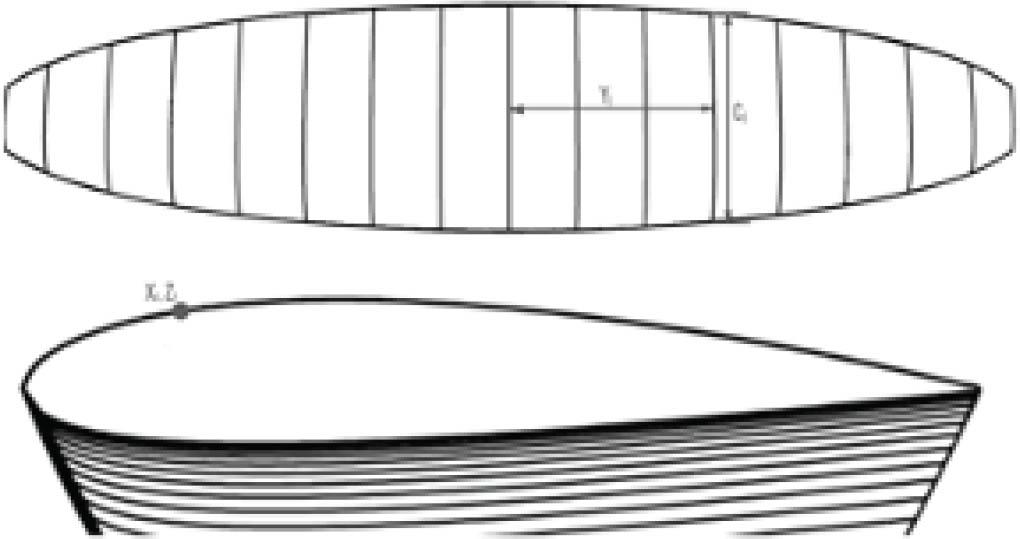Geometrical characteristics of the wing contour and the dimensionless coordinates of the airfoil (“j” concerns the subsequent rib in the XY plane; “i” denotes the coordinates in the XZ plane).

Next, 23 dimensionless coordinates that form the basic airfoil profile are defined on the circumference of the cross section on the XZ plane (Figure 1). The coordinates of an optional point in the 3D space coordinate system are obtained from the elliptic equation in the following form: $x=xiCj+12(C0−Cj);y=(R+zjCj)sin(yjR);z=[(R+zjCj)cos(yjR)]−R.$x = {x_i}{C_j} + {1 \over 2}({C_0} - {C_j});y = (R + {z_j}{C_j})\sin \left( {{{{y_j}} \over R}} \right);z = \left[ {(R + {z_j}{C_j})\cos \left( {{{{y_j}} \over R}} \right)} \right] - R.

The coordinates of an optional point on the wing contour are determined by a simple program in the MatLab environment. These points create the wing geometry in the ANSYS Design Modeler [11, 12]. The contour is smoothed using the spline curves, and the surface of paraglider wing is created using the skin/loft option. The thickness of the covering material is assumed equal to 2 mm and applied with the thin tool. However, the change in thickness necessitates the application of Porous Media Model in models of the given air permeability.

Creation of finite volume mesh and solution in ANSYS Fluent

The computational domain of the wing geometry is a cuboid including a half of the paraglider structure. On the symmetry plane, the Symmetry boundary condition is used. The dimensions of the cuboid are 20 m in front and 40 m in other directions. Its external planes are subjected to the surrounding conditions (T = 300 K; p = 101,325 Pa) and approximated by the condition Pressure Far-Field. The external surfaces of the contour are defined by the Interior boundary condition and the Porous Media Model.

A computational mesh is created for the extruded objects (i.e., the paraglider walls and the air inside the contour) using the procedure Sweep Method. The program generates a surface mesh on the so-called Source plane, which is the cross section of wing surface. Next, the mesh topology of the source plane is extended along the object. The volume cells are perpendicular to the wing surface and divided into the corresponding planes. Along the span are applied 80 segments, and the dividing planes are the most distant around the middle of the profile. The distances between the dividing planes decrease toward the wing tip.

The mesh is determined by the prescribed number of nodes on each edge, and the tool used is Edge Sizing. Each segment defining the upper and lower contours is divided into 30 parts, whereas the segments contacting the paraglider surface and the inlet contour are divided into three parts.

The air inside the contour is approximated in the plane of symmetry by the cells of the prescribed volumes. The applied procedure Face Sizing does not determine the cell's dimensions. The surrounding air is approximated by the unstructured computational mesh made of tetrahedron elements, and the tool is Tetrahedrons Patch Conforming Method.

Therefore, the mesh is made of structural cells (the wing surfaces, and the air inside the wing contour) and nonstructural elements (the surrounding air) . The quality parameters are acceptable: the parameter Orthogonal Quality = 0.76 (the perfect value =1.0), and Skewness = 0.25 (the perfect value =0). Structural elements are preferred in computer fluid dynamics (CFD) because of their accurate results and stable calculations. On the other hand, the structural mesh is difficult to create in the case of complicated geometry.

The nodes of two adjacent meshes (the structural net inside the wing contour and the non-structural in surrounding air) are incompatible on the external surface of the wing, which is the critical disadvantage. However, the procedure nonconformal mesh interface is weak, and the results obtained are inadequate for 2D cases . It is necessary to equalize the mesh dimensions using Make Polyhedra tool  and convert the tetrahedral mesh to a polyhedral one (Figure 2). The transfer into ANSYS Fluent creates cells of larger dimensions, similar to the cubic cells discretizing the wing. The number of elements is reduced to 121,740, which cuts down the calculation time.

The turbulence model Spalart–Allmaras is the most adequate because of the low Reynolds number . The fluid filling the computational area is air/ideal gas (Materials → air → ideal gas). The wing surface of the prescribed permeability is described by the boundary condition Cell zone conditions → Porous Media.

The permeability is defined using the parameter Porous Resistance from the pressure gradient equation .

$1β=−Δpt×μ×vm${1 \over \beta} = {{- \Delta p} \over {t \times \mu\times {v_m}}}

Information about the selected materials and parameters are shown in Table 1.

Values of the Porous Resistance parameter for corresponding materials, based on Korycki and Szafrańska 

FabricPermeability$[1m2⋅min]$[{1 \over {{m^2} \cdot min}}]under 2,000 PaVm [m/s]1/β [1/m2]
Dominico Tex DMF 2060.000102.236 × 1015
Porcher Marine 9017 PU E38A400.000678.385 × 1013
Skytex 36 after flutter and hydrolysis2000.003331.677 × 1013

The basic control parameter of stable calculations is the Courant number (Cn), determining the time step size for the given cell geometry and velocity in the unit cell. The calculations started with a small Courant number (Cn = 2) to control the process stability. The calculations are stable, and the number grows to Cn = 10; the time step increases gradually. Permeable textiles need increased number of iterations than an impermeable material , i.e., 1,000–1,500 iterations. It is forced by the variable conditions and complicated mesh.

Numerical results and discussion

According to Figure 3a and Table 2, the best aerodynamic characteristics result from the textile fabric of the lowest permeability (the mean pressure inside the wing p = 101,415.72 Pa; the ratio of lift coefficient by the drag coefficient [Cl/Cd] = 12.288). The covering fabric Porcher Marine 9017 PU E38A (used 20 years ago) presents apparently worse results . However, the wing remains safe and stable during the flight. Its Cl/Cd ratio is 0.6627, lower than the Cl/Cd ratio for Dominico Tex DMF 20.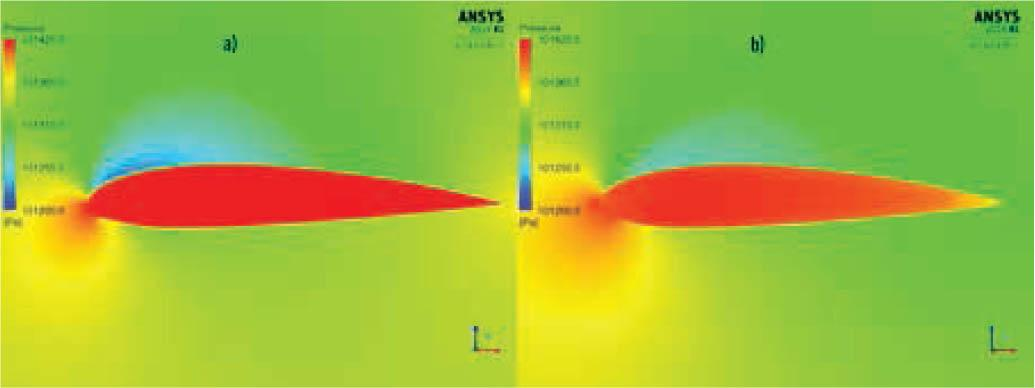Pressure distribution in the plane of symmetry (α = 6°; v=45 km/h): (a) wing made of Dominico Tex DMF 20; and (b) wing made of Skytex 36 after flutter and hydrolysis.

Example of interpretation of numeric values obtained in the course of numerical calculations (α = 6°; v = 45 km/h)

ParameterDominico Tex DMF 20Porcher Marine 9017 PU E38ASkytex 36 (after flutter and hydrolysis)
Average pressure inside paraglider wing101,415.72 Pa101,412.6 Pa101,398.35 Pa
Mass flow rate0.251 kg/s1.435 kg/s5.8542 kg/s
Lift force1,091.045 N1,085.883 N812.2437 N
Drag force88.7882 N93.4068 N199.7884 N
Cl/Cd ratio12.28811.62534.0655

Cl/Cd, ratio of lift coefficient by the drag coefficient.

The paraglider covered with Skytex 36 after flutter and hydrolysis is probably unable to fly. The uniform overpressure on the outer side of the leading edge probably disturbs the creation of overpressure inside the contour (Figure 3b). To verify the results, let us compare the pressure distribution on the upper and lower wing surfaces (Figure 4) and the values in Table 2. The increase in pressure on the leading edge is around 101,405 Pa, and the mean pressure inside the contour is 101,398.35 Pa (compatible with Figure 3b). The values are nearly comparable, so the flight is either impossible or unstable and extremely dangerous.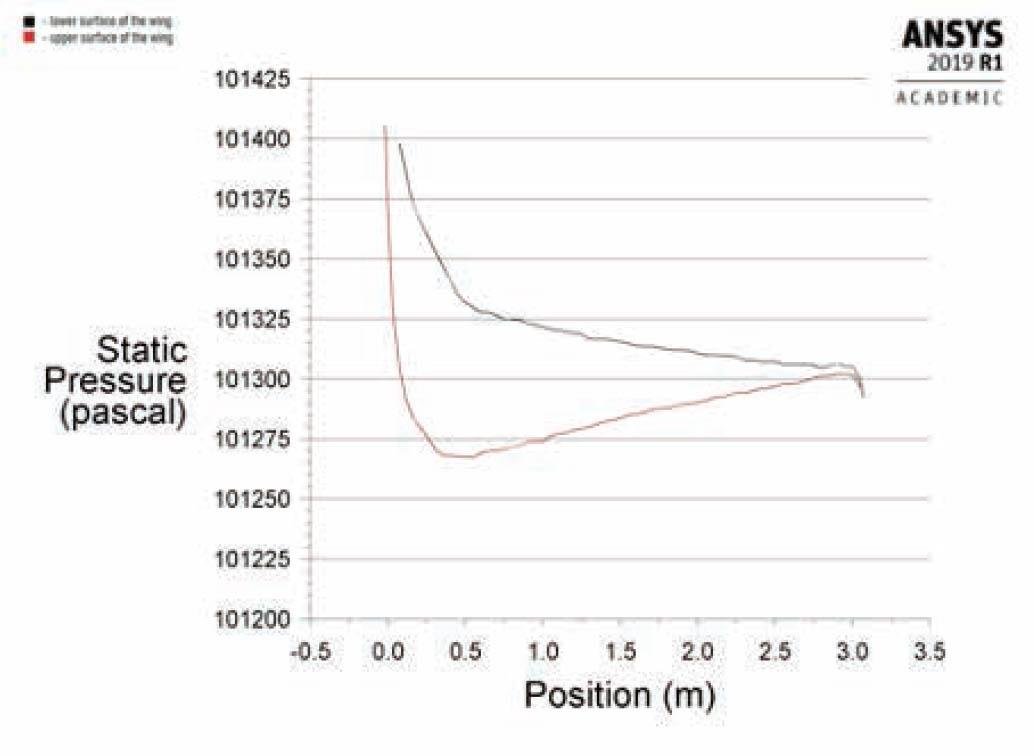Pressure on the upper surface (black line) and the lower surface (red line) of the paraglider wing (wing covered with the SKYTEX 36 after flutter and hydrolysis; α = 6°; v = 45 km/h).

The covering materials with the lowest air permeability are subjected to the highest internal forces during the flight because of the highest pressure inside the wing. Therefore, the further calculations of forces transmitted through the seams necessitate a covering material of the lowest permeability. The pressure distribution is next analyzed for the angle α = 6° and the velocities v = 30, 33, 36, 39, 42, and 45 km/h. The internal forces in the textile covering including seams are indicated by the difference between the maximal pressure inside the contour and the minimal pressure outside the material. The difference is equal to 79 Pa for the lowest velocity (Figure 5a) and 187 Pa for higher velocities.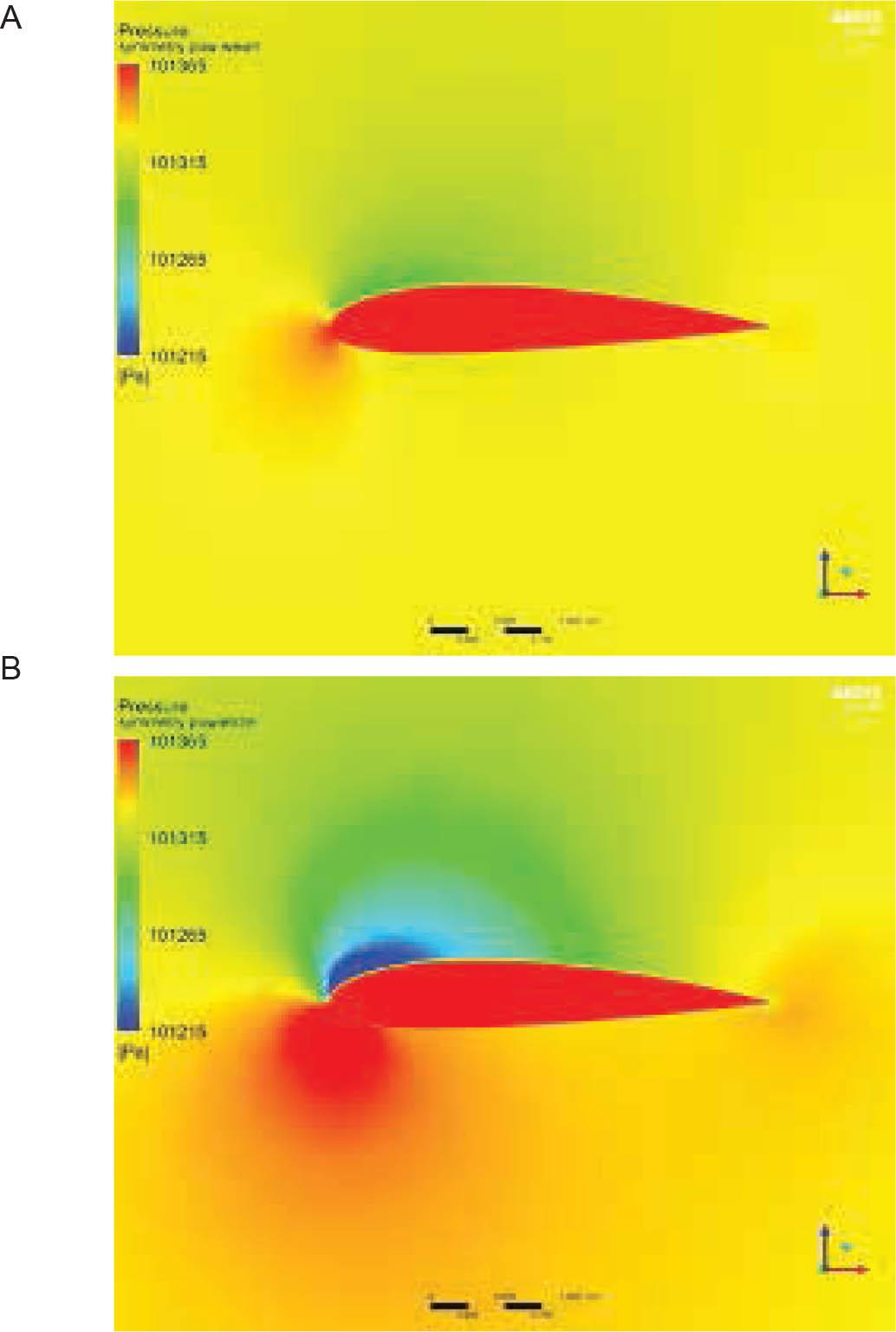Pressure distributions for the wing made of Dominico Tex DMF 20: (a) velocity 30 km/h, angle of attack 6°; and (b) velocity 45 km/h, angle of attack 12°.

The Cl/Cd ratio = Pz/Px (the ratio of the lift force to the drag force) is not particularly sensitive to velocity change; the maximal difference is equal to 0.008 (Figure 6b). The higher the velocity, the better is the aerodynamic characteristic. The velocity equal to v = 33–36 km/h causes the Cl/Cd ratio to decrease slightly. The reasons are the vortices at the trailing edge caused by the increasing speed.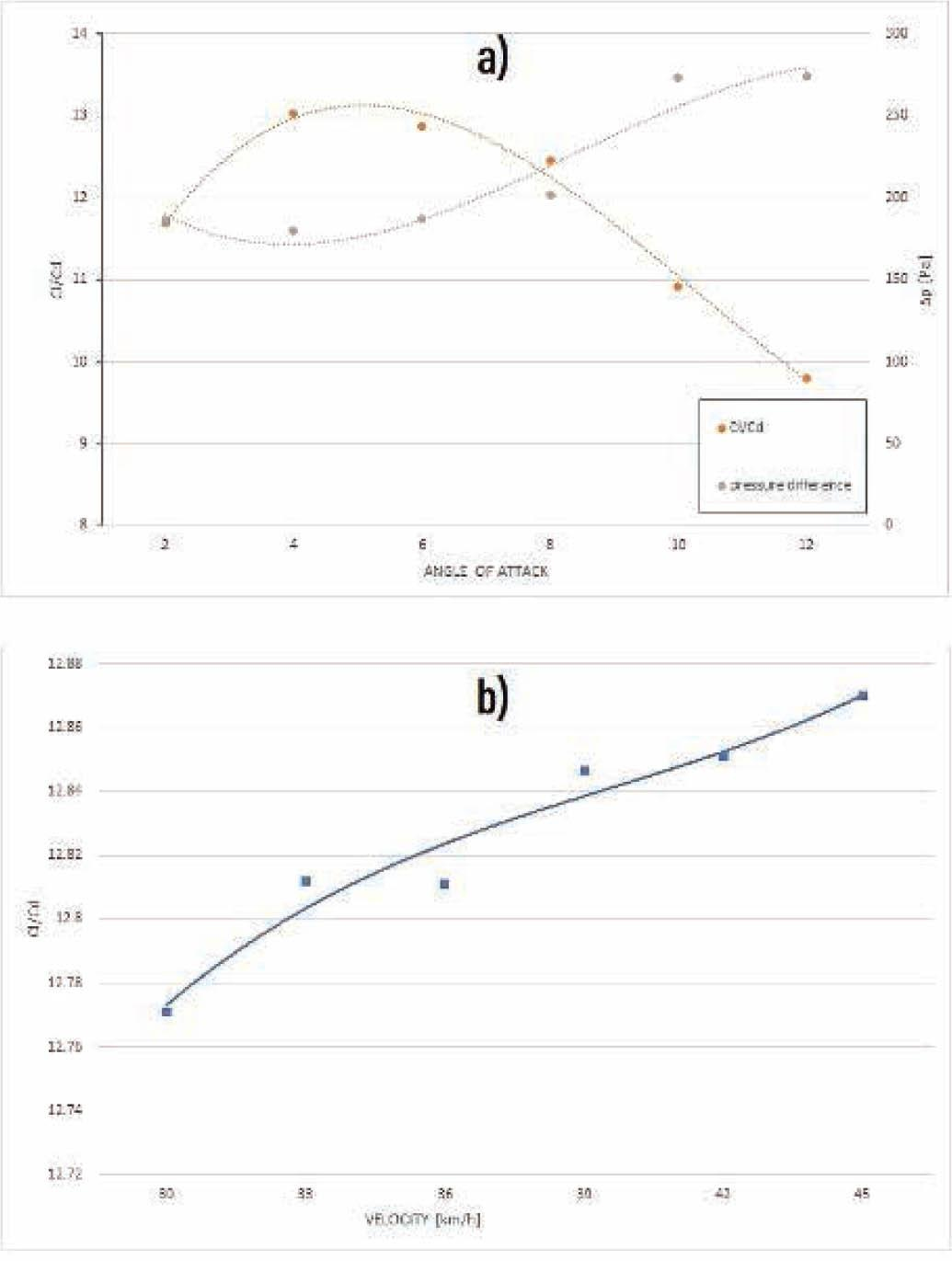(a) Sensitivity of Cl/Cd and the maximal pressure difference ratio to the angle of attack (v = 45 km/h). (b) Sensitivity of Cl/Cd to velocity (a = 6°). Wing is covered with the Dominico Tex DMF 20 material.

Let us now determine the angles of attack that provide the optimal and the most extreme conditions of flight. They are essential to define the pressure inside the wing contour as the measure of seam load. The distribution of forces is analyzed for the typical velocity v = 45 km/h and the angles of attack α = 2, 4, 6, 8, 10, and 12o. The angle tending to zero causes the aerodynamic lift P to be nearly vertical (Figure 7). The larger the angle of attack, the greater is the drag force because of the rapidly growing aerodynamic coefficient. The optimal angle of attack in the context of both flight stability and pressure distribution is α = 6°. The optimal angle is confirmed by the Cl/Cd ratio = Pz/Px, which is the maximal lift force related to the drag force. The best aerodynamic characteristics are defined by the maximal Cl/Cd ratio for the angle α = 4–6°. However, the flight is more stable for the maximal acceptable value α = 6°. Summarizing, the optimal flight parameters assumed during the analysis are α = 6°; v = 45 km/h.

The biggest internal forces are caused by the most extreme conditions in relation to the angle of attack, i.e., the difference between the maximal pressure inside and the minimal pressure outside the covering (Figure 6a). The pressure difference is not sensitive to the variable velocity. Thus, the extreme flight parameters that indicate the biggest internal forces of the wing material, including seams, are the following α = 12°; v = 45 km/h (Figure 5b).

Seams in paraglider wing

The physical model of seam rupture is complicated and unpredictable in the context of four phenomena: (i) the force to seam rupture is differently directed because of different pressures inside the contour; (ii) the dynamic conditions during the flight and tests generate different directions and values of the friction force; (iii) the frictional force between the thread and the fabric is variable; and (iv) the thread is subjected to elongation, which causes variable pressure and friction inside the seam.

Thus, the detailed parameters that interact with the test results and seam load are the following: (i) the mean pressure inside the wing covered with material of the lowest permeability (p = 101,414 Pa); and (ii) the difference Δp between the maximal pressure inside (pmax) and the minimal pressure outside (pmin) the material of the lowest permeability (Δp = pmaxpmin = 274 Pa).

The force transmitted through the paraglider seams can be calculated by the accurate method, i.e., introducing the elementary forces in the textile covering and connecting seams (Figure 8). Let us apply a rectangular piece of material between the first and second joints of the dimensions Y1 and C0. This part of the material is the largest and transmits the maximal pressure load. The segment Y1 corresponds to the distance between adjacent joints in the center of wing, whereas C0 corresponds to the longest paraglider chord (Figure 1). The rectangular sample is attached with seams on both sides with a length of C0. The force caused by the pressure difference Δp = pmaxpmin inside the wing covering can be expressed as follows: $Ftotal=∫sΔp(x,y)dS=∬xyΔp(x,y)dxdy.${F_{{\rm{total}}}} = \int\limits_s {\Delta p(x,y)dS = \int\!\!\!\int\limits_{xy} {\Delta p(x,y)dxdy}}.

The pressure difference is constant in the infinitesimal time step and the integral is simplified as follows: $Ftotal=Δp(x,y)∫sdS=(pmax−pmin)S=(pmax−pmin)C0Y1.${F_{{\rm{total}}}} = \Delta p(x,y)\int\limits_s {dS = ({p_{\max}} - {p_{\min}})S = ({p_{\max}} - {p_{\min}}){C_0}{Y_1}}.

On the other hand, the force caused by the pressure difference is balanced by the internal force in the seams, denoted by the formula: $Ftotal=∫Isew1fsew1(x)dx+∫Isew2fsew2(x)dx${F_{total}} = \int\limits_{{I_{sew1}}} {{f_{sew1}}(x)dx}+ \int\limits_{{I_{sew2}}} {{f_{sew2}}(x)dx} where fsew1 and fsew2 are the forces per unit length on the first and second joints/seams; lsew1 and lsew2 are the lengths of adequate seam. Assuming the constant forces fsew1 = fsew2 = fsew along the seam and the rectangular shape of material, we obtain the following from Eq. (6): $Ftotal=fsew1∫Isew1dx+fsew2∫Isew2dx=2fsewIsew=2fsewC0${F_{{\rm{total}}}} = {f_{sew1}}\int\limits_{{I_{sew1}}} {dx + {f_{sew2}}} \int\limits_{{I_{sew2}}} {dx = 2{f_{sew}}{I_{sew}} = 2{f_{sew}}{C_0}}

Comparing Eq. (5) and (7), the force acting on the seams per unit of length has the following form: $Fseam=0.5(pmax−pmin)Y1${F_{{\rm{seam}}}} = 0.5({p_{\max}} - {p_{\min}}){Y_1}

Introducing the dimensions of the paraglider's wing, the force is Fseam = 45.2 N/m.

On the other hand, the sample teared during the tests is short, and the approximate force is of the order of Fseam = 10–15 N.

Evaluation of the maximal force to seam rupture and the relative elongation during rupture

Let us evaluate the seams prepared along both warp thread and weft thread directions of the fabrics Dominico Tex 20 DMF and Dominico Tex 30 DMF (Table 3). The sewn seams applied to connect the covering elements are made according to International Organization for Standardization (ISO) 4916 [15, 16], and the seam number is 1.01.03/301.301 (Figure 9). The sewing conditions are the following: (i) the sewing threads – made of 100% polyester; (ii) the needle size and finish – Schmetz R 90 nickel plated; (iii) the stitch type – quilted seam 301; (iv) the stitch density – 3/cm; and (v) the test samples – prepared using the industrial sewing machine Siruba.

Characteristics of textile material tested; and the fabric applied on the bottom surface of the wing

NameRaw material compositionSquare mass S, g/m2Thickness h, mmType of weaveNumber of threads/dmMaximum force P, NRelative elongation ε, %
Dominico Tex 20 DMFPA 6.6 100% + impregnant PU on the left-hand side of material340.05RipstopWarp: 616; weft: 668Warp: 340; weft: 338Warp: 22; weft: 25
Dominico Tex 30 DMFPA 6.6 100% + impregnant PU on the left-hand side of material370.07RipstopWarp: 504; weft: 508Warp: 400; weft: 432Warp: 21; weft: 25

PA, polyamide; PU, polyurethane.

The seam quality index is determined by (i) the maximal force to seam rupture F (in Newtons) and (ii) the relative elongation during rupture ε(in percentage). The seam indexes are analyzed considering the directions of acting force and seam: (i) the parallel direction (the longitudinal direction) and (ii) the perpendicular direction (the cross direction) (Figure 10).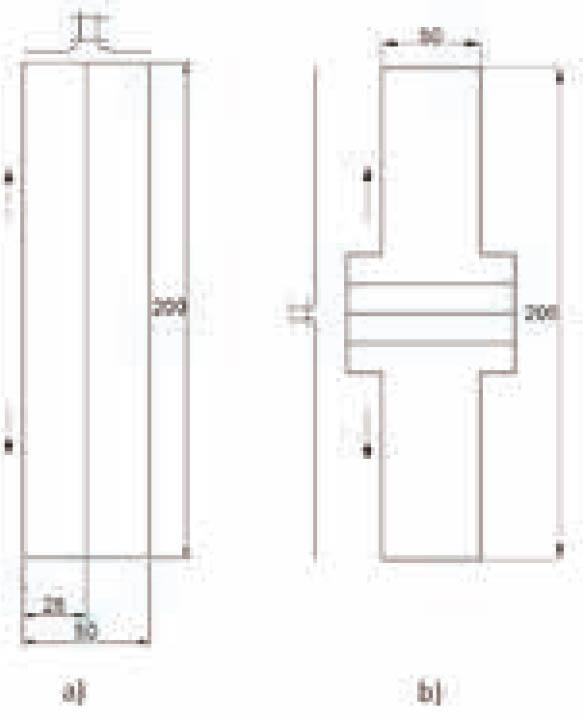Technological project of sewn seams. Determination of seam strength oriented parallel and perpendicular in relation to the direction of acting force: (a) longitudinal seam and (b) transverse seam.

The strength of seams is tested using the testing machine INSTRON Series 5560, provided with the computer program of TX series. Samples of seams were subjected to tension within the distance equal to 100 mm between clamps of testing machine at the speed of load increase of 50 mm/min. Before testing, all samples were conditioned at standard conditions, i.e., relative humidity of 65% and temperature of 20°C. The same method was applied for both seams and fabric.

The test program includes the mean results obtained for the seams prepared along both warp thread and the weft thread directions. Analysis of bidirectional tests is significant because the seams are subjected to different loads during the normal flight, i.e., longitudinal and cross directions.

The maximal force to seam rupture depends on the warp/weft direction and longitudinal/cross orientation of the acting force (Figure 10). The differences are substantial (Figure 11). Measuring in the warp direction, the force is bigger for the longitudinal than the cross direction (490N/194N for Dominico Tex 20 DMF, 577N/331N for Dominico Tex 30 DMF). The corresponding values in the weft direction are equal to 600N/172N (Dominico Tex 20 DMF) and 581N/210N (Dominico Tex 30 DMF). The tearing of fabric in the cross direction discontinues the test. The sample is tested using two methods, the only difference being the shape of sample. The fabric is always torn, and the seam remains still intact (Figure 12).

The relative elongation during rupture is almost comparable. Some differences exist in the warp direction for both fabrics (21%/23.3% for Dominico Tex 20 DMF, and 23%/27% for Dominico Tex 30 DMF). The uniform elongation of textile coverage in all directions is advantageous during the flight of paraglider.

Considering the quality index and theoretical calculations, the seams connecting the textile components made of Dominico Tex fabric are resistant to pressure inside the wing contour. Additionally, the factor of safety is relatively high. The Dominico Tex fabric is commonly used to cover the paraglider. Moreover, there is no information available about accidents resulting from tearing of the seams during the flight.

Conclusions

The paraglider wing is a complex structure subjected to different loads in dynamic conditions and various phenomena during the flight. Seams connecting the textile elements are the most loaded component of the wing, so it they should be resistant. The difficulty is a complicated physical description of seams, that which precludes the unequivocal formulation of the physical model. Thus, the only method left is to compare the theoretical force caused by the maximal pressure difference on the material surface with the seam quality index formulated during the tests. The mean pressure inside the contour remains a reference level of the load.

The mean pressure inside the wing and the maximal difference between the pressure on the inner and outer surfaces are determined using the CFD with the Porous Media tool. The sensitivities of aerodynamic characteristics during the flight are analyzed in the context of the material properties, the velocity, and the angle of attack. The state parameter of seam estimation is the maximal pressure difference between the surfaces of the wing contour, which interacts with the test results. Theoretical considerations allow the determination of the maximal predicted force to seam rupture inside the biggest fragment of textile covering.

The seam quality index is determined by the maximal force to rupture and the relative elongation during rupture. The forces in the warp/weft direction and longitudinal/cross orientation are quite different, which result in textile tearing. The relative elongation during rupture is comparable. The correct flight of the paraglider wing and the lack of available information about accidents caused by the tearing of seams indicate that the seams subjected to the current pressure inside the contour are resistant enough.

The interaction between the CFD/ANSYS Fluent and the tests of seam quality index seems to be a useful tool to assess the paraglider wing, its behavior, and safety during the flight.

In the future, we intend to formulate the physical model of the seam subjected to variable dynamic loads. Theoretical considerations, description of the physical model, and tests of the seams should be introduced for other types of textiles.

#### Values of the Porous Resistance parameter for corresponding materials, based on Korycki and Szafrańska 

FabricPermeability[1m2min][{1 \over {{m^2} \cdot min}}]under 2,000 PaVm [m/s]1/β [1/m2]
Dominico Tex DMF 2060.000102.236 × 1015
Porcher Marine 9017 PU E38A400.000678.385 × 1013
Skytex 36 after flutter and hydrolysis2000.003331.677 × 1013

#### Characteristics of textile material tested; and the fabric applied on the bottom surface of the wing

NameRaw material compositionSquare mass S, g/m2Thickness h, mmType of weaveNumber of threads/dmMaximum force P, NRelative elongation ε, %
Dominico Tex 20 DMFPA 6.6 100% + impregnant PU on the left-hand side of material340.05RipstopWarp: 616; weft: 668Warp: 340; weft: 338Warp: 22; weft: 25
Dominico Tex 30 DMFPA 6.6 100% + impregnant PU on the left-hand side of material370.07RipstopWarp: 504; weft: 508Warp: 400; weft: 432Warp: 21; weft: 25

#### Example of interpretation of numeric values obtained in the course of numerical calculations (α = 6°; v = 45 km/h)

ParameterDominico Tex DMF 20Porcher Marine 9017 PU E38ASkytex 36 (after flutter and hydrolysis)
Average pressure inside paraglider wing101,415.72 Pa101,412.6 Pa101,398.35 Pa
Mass flow rate0.251 kg/s1.435 kg/s5.8542 kg/s
Lift force1,091.045 N1,085.883 N812.2437 N
Drag force88.7882 N93.4068 N199.7884 N
Cl/Cd ratio12.28811.62534.0655

Maślanka, P., Korycki, R. (2019). Textile cover effect on aerodynamic characteristics of a paraglider wing. Fibres Textiles in Eastern Europe, 27, 133(1), 78–83.MaślankaP.KoryckiR.2019Textile cover effect on aerodynamic characteristics of a paraglider wingFibres Textiles in Eastern Europe, 271331788310.5604/01.3001.0012.7511Search in Google Scholar

Korycki, R. (2011). Modeling of transient heat transfer within bounded seams. Fibres Textiles in Eastern Europe, 19, 5(88), 112–116.KoryckiR.2011Modeling of transient heat transfer within bounded seamsFibres Textiles in Eastern Europe, 19588112116Search in Google Scholar

Korycki, R., Szafrańska, H. (2017). Thickness optimisation of sealed seams in respect of insulating properties. Fibres and Textiles in Eastern Europe, 25, 2(122), 68–75.KoryckiR.SzafrańskaH.2017Thickness optimisation of sealed seams in respect of insulating propertiesFibres and Textiles in Eastern Europe, 252122687510.5604/12303666.1228185Search in Google Scholar

Korycki, R., Szafranska, H. (2016). Thickness optimisation of textiles subjected to heat and mass transport during ironing. Autex Research Journal, 16(3), 165–174.KoryckiR.SzafranskaH.2016Thickness optimisation of textiles subjected to heat and mass transport during ironingAutex Research Journal16316517410.1515/aut-2015-0035Search in Google Scholar

Korycki, R., Szafrańska, H. (2016). Optimisation of pad thicknesses in ironing machines during coupled heat and mass transport. Fibres and Textiles in Eastern Europe, 24, 1(115), 128–135.KoryckiR.SzafrańskaH.2016Optimisation of pad thicknesses in ironing machines during coupled heat and mass transportFibres and Textiles in Eastern Europe, 24111512813510.5604/12303666.1172095Search in Google Scholar

Korycki, R. (2007). Shape optimization and shape identification for transient diffusion problems in textile structures. Fibres Textiles in Eastern Europe, 15, 1(60), 43–49.KoryckiR.2007Shape optimization and shape identification for transient diffusion problems in textile structuresFibres Textiles in Eastern Europe, 151604349Search in Google Scholar

Korycki, R., Szafrańska, H. (2013). Modelling of temperature field within textile inlayers of clothing laminates. Fibres Textiles in Eastern Europe, 21, 4(100), 118–122.KoryckiR.SzafrańskaH.2013Modelling of temperature field within textile inlayers of clothing laminatesFibres Textiles in Eastern Europe, 214100118122Search in Google Scholar

Puszkarz, A. K., Korycki, R., Krucinska, I. (2016). Simulations of heat transport phenomena in a three-dimensional model of knitted fabric. Autex Research Journal, 16(3), 128–137.PuszkarzA. K.KoryckiR.KrucinskaI.2016Simulations of heat transport phenomena in a three-dimensional model of knitted fabricAutex Research Journal16312813710.1515/aut-2015-0042Search in Google Scholar

Frącczak, Ł., Rafał, D., Piotr, Z., Matusiak, M. (2019). Parameterization of seersucker woven fabrics using laser techniques. Autex Research Journal, 19(3), 243–249.FrącczakŁ.RafałD.PiotrZ.MatusiakM.2019Parameterization of seersucker woven fabrics using laser techniquesAutex Research Journal19324324910.1515/aut-2018-0042Search in Google Scholar

Matusiak, M., Wilk, E. (2018). Investigation of mechanical and utility properties of two-layer cotton woven fabrics. Autex Research Journal, 18(2), 192–202.MatusiakM.WilkE.2018Investigation of mechanical and utility properties of two-layer cotton woven fabricsAutex Research Journal18219220210.1515/aut-2017-0032Search in Google Scholar

Anonymus. (2010). ANSYS fluent user’s guide. ANSYS, Inc. (Canonsburg, U.S.A.).Anonymus2010ANSYS fluent user’s guideANSYS, IncCanonsburg, U.S.A.Search in Google Scholar

Anonymus. (2010). Introduction to ANSYS meshing. ANSYS, Inc. (Canonsburg, U.S.A.).Anonymus2010Introduction to ANSYS meshingANSYS, Inc.Canonsburg, U.S.A.Search in Google Scholar

Kudela, H. (2016). Introduction to the laboratory of computational fluid dynamics (in Polish). Wrocław University of Technology.KudelaH.2016Introduction to the laboratory of computational fluid dynamics (in Polish)Wrocław University of TechnologySearch in Google Scholar

Anonymus. Web site: http://www.porcher-sport.com/fr/.AnonymusWeb site: http://www.porcher-sport.com/fr/Search in Google Scholar

Anonymus. Web site: https://www.coats.com/en/Information-Hub/Finding-the-Best-Seam-Type.AnonymusWeb site: https://www.coats.com/en/Information-Hub/Finding-the-Best-Seam-Type.Search in Google Scholar

ISO 4916:1991. Textiles — Seam types — Classification and terminology.ISO 49161991Textiles — Seam types — Classification and terminologySearch in Google Scholar

• #### Archaeology and Virtual Simulation Restoration of Costumes in the Han Xizai Banquet Painting

Empfohlene Artikel von Trend MD